Example Questions

1 2 3 4 6 Next →

Example Question #15 : How To Find The Volume Of A Tetrahedron

What is the volume of a regular tetrahedron with an edge length of 6?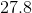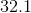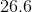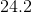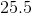Explanation:

The volume of a tetrahedron can be solved for by using the equation: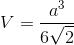whereis the measurement of the edge of the tetrahedron.

This problem can be quickly solved by substituting 6 in for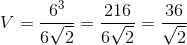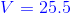Example Question #16 : How To Find The Volume Of A Tetrahedron

What is the volume of the tetrahedron shown below?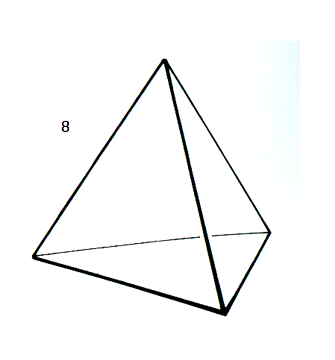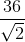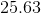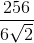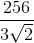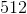Explanation:

The volume of a tetrahedron is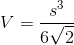.

This tetrahedron has a side with a length of 8.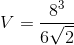, which becomes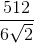.

You can reduce that answer further, so that it becomes1 2 3 4 6 Next →